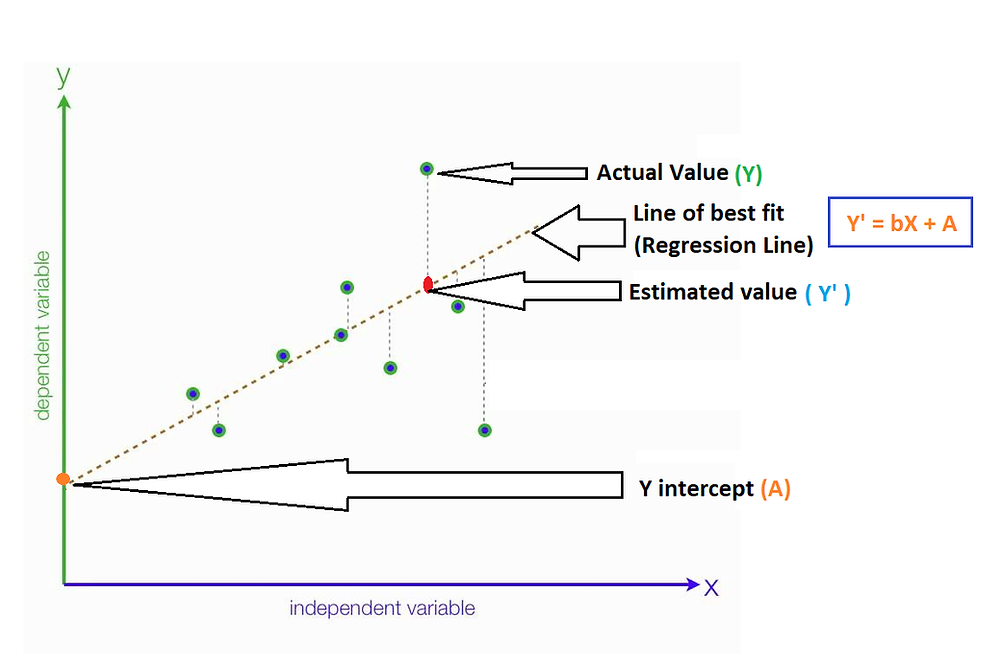Search

# What is “Line of Best fit” in linear regression?

Simple linear regression is a statistical method that allows us to summarize and study relationships between two variables: One variable is the predictor, explanatory, or independent variable and the other one is the dependent variable.

Linear Regression is the process of finding a line that best fits the data points available on the plot, so that we can use it to predict output values for given inputs.

So, what is “Best fitting line”?

A Line of best fit is a straight line that represents the best approximation of a scatter plot of data points. It is used to study the nature of the relationship between those points.

The equation to find the best fitting line is:

Y` = bX + A

where, Y` denotes the predicted value

b denotes the slope of the line

X denotes the independent variable

A is the Y interceptOn a chart, a given set of data points would appear as scatter plot, that may or may not appear to be organized along any line. It is possible to draw many straight lines through the data points in the chart, but to find a line of best fit that minimizes the distance of those points from that line is one of the most important outputs of regression analysis.

So, how do we find a line of best fit using regression analysis?

Usually, the apparent predicted line of best fit may not be perfectly correct, meaning it will have “prediction errors” or “residual errors”.

Prediction or Residual error is nothing but the difference between the actual value and the predicted value for any data point. In general, when we use Y` = bX +A to predict the actual response Y`, we make a prediction error (or residual error) of size:

E = Y – Y`

where, E denotes the prediction error or residual error

Y` denotes the predicted value

Y denotes the actual value

A line that fits the data "best" will be one for which the prediction errors (one for each data point) are as small as possible.

The below diagram depicts the simple representation with all the above discussed values:Regression analysis uses “least squares method” to generate best fitting line. This method builds the line which minimizes the squared distance of each point from the line of best fit.

I will talk about “least squares method” in detail with an example in my next blog.

To summarize:

• The Line of Best Fit is used to express a relationship in a scatter plot of different data points.

• It is an output of regression analysis and can be used as a prediction tool.